# Darcian Velocity and Temperature Jump Effects on Convection from Vertical Surface Embedded in Porous Media

Darcian Velocity and Temperature Jump Effects on Convection from Vertical Surface Embedded in Porous Media

V.M. Al-khliefat* H. M. Duwairi

Mechanical Engineering Department, Faculty of Engineering and Technology, University of Jordan, 11942 Amman, Jordan

Corresponding Author Email:
DUWAIRI@JU.EDU.JO
Page:
97-102
|
DOI:
https://doi.org/10.18280/ijht.330216
N/A
|
Accepted:
N/A
|
Published:
30 June 2015
| Citation

OPEN ACCESS

Abstract:

In this work the temperature jump effects on convection from vertical plate embedded in porous media using Darcy model has been investigated numerically. The governing equations: continuity, Darcy law and energy were transformed into dimensionless form using a set of suitable variables then solved numerically using a finite difference method. Results obtained were graphically interdicted to illustrate the effects of temperature jump on the near plate fluid velocity, temperature, shear stress, and Nusselt number. It was found that the increasing of temperature jump parameter reduced velocity and heat transfer rates between surface and fluid for both natural and mixed convection. It was also found that the temperature jump does not affect the rate of forced convection heat transfer.

Keywords:

Darcian velocity, Temperature jump, Convection, Porous media.

1. Introduction

Transport processes through porous media play important roles in diverse applications, such as in geothermal operations, petroleum industries, thermal insulation, design of solid-matrix heat exchangers, chemical catalytic reactors, and many others. The study of convection heat transfer and fluid flow in porous media has received great attention in recent years. Due to the wide range of applications on convection heat transfer in porous media, many studies had been carried out on this topic. Most of these studies concentrated on no temperature jump between the surface and the fluid.

Bejan and Poulikakos , used Forchheimer’s model to study vertical boundary layer natural convection in a porous medium. They reported similarity solutions for vertical boundary layer natural convection near a solid wall adjacent to a fluid-saturated porous medium. It was shown that at high pore Reynolds number Darcy flow model is no longer valid. It was shown that the heat transfer results totally differ from those obtained from traditional Darcy flow researchers. Chien et al.  performed an analysis for mixed convective flow through a saturated porous medium adjacent to a vertical surface with the heating condition of power-law variation in the wall temperature. In modeling the flow through porous media, no-jump temperature boundary condition, high-flow-rate inertia forces, thermal dispersion and near- wall porosity variation were investigated.  Transformed system of equations was solved by finite- difference method. Numerical results showed that non- Darcian and thermal dispersion effects have important effects on temperature and velocity profiles and rates of heat transfer from the vertical plate. Thus, recent studies investigated this condition in determination of heat transfer rate. Al-Badawi and duwairi  and Shehadah and Duwairi  studied convection heat transfer in internal flows. in James and Davi  studied the flow in the interfacial region of an open porous medium by solving Stokes model for flow in a channel partially filled with an array of circular cylinders beside one wall. The cylinders were oriented across the flow and widely spaced, therefore the solid volume fraction was 0.1 or less. Cao and Baker  developed Laminar mixed convection model over an isothermal vertical plate with ﬁrst-order momentum and thermal discontinuities at the wall. Numerical solutions were obtained for various conditions. Velocity and temperature profiles within the boundary layer were introduced. The effect of non-continuum upon temperature jump, slip velocity, wall shear stress and boundary layer thickness in both liquid and gaseous flow was illustrated. Yazdi et al  studied convective heat transfer of the slip liquid flow over horizontal plate within the porous media at constant heat flux boundary conditions. Their model assumed the fluid has a slip boundary condition on the surface. The governing equations of the boundary layer were transformed into ordinary differential equations and solved numerically using a fourth order Runge-Kutta and shooting method. It was shown that the velocity adjacent to the wall is increased by both suction and slip coefficient. Bhattacharyya et al  analyzed the boundary layer forced convective flow and heat transfer of an incompressible fluid over a porous plate embedded in a Darcy porous medium. Velocity and thermal slips at the boundary were taken into account. They found that the increase of velocity slip parameter reduces the momentum boundary layer thickness and enhances the heat transfer from the plate; on the other hand, heat transfer decreases with thermal slip parameter. Bhattacharyya  considered velocity and mass slips at the boundary in the analysis of the steady boundary layer flow and mass transfer with nth order chemical reaction past a porous plate embedded in a Darcy porous medium. The reduced nonlinear equations were solved numerically. It was found that the increase of velocity slip reduces the momentum boundary layer thickness and enhances the mass transfer. Miguel  used Boltzmann equation method to study non-Darcy flow in porous media. Darcy-Forchheimer equation was investigated and correlations for macroscopic transport properties were introduced (permeability and the inertial parameter). This work covered both no-slip and the slip-flow regimes. Jashim Uddin et al  studied two dimensional steady laminar boundary layer flow of a nano-fluid past vertical plate heated with linear momentum slip boundary condition. The governing equations with boundary conditions were solved numerically by Maple 13. It was found that the skin friction coefficient is reduced with the momentum slip and the buoyancy ratio parameters. On the other hand, it is enhanced with the convective heat transfer parameter, and that mass transfer rate is enhanced with the Lewis number and the convective heat transfer parameter falls with the thermophoresis parameter. Mishra  investigated the steady mixed convection flow between two vertical plates filled with porous materials with slip velocity and temperature jump. The analytic solutions for the velocity and temperature profiles had been obtained for different values of the Rayleigh number, Darcy number. Njael and Makinde  discussed the effect of magnetic field on boundary layer flow of an incompressible electrically conducting water-based nanofluid over a heated vertical porous plate with slip boundary condition. Graphical results were presented and discussed considering the effect of dominant parameters (solid volume fraction, buoyancy effect, Eckert number, suction/injection parameter, Biot number, and slip parameter) on the dimensionless velocity, temperature, magnetic field parameter skin friction coefficient, and heat transfer rate.

In this work the slip velocity and temperature jump effects on forced, natural and mixed convection heat transfer problem from vertical surfaces embedded in saturated porous medium are investigated. The governing equations; continuity, momentum and energy with the corresponding slip velocity and temperature jump boundary conditions for different convection heat transfer problems are going to be written in suitable dimensionless from, then solved using suitable finite difference technique. Different velocity profiles, temperature profiles, coefficient of friction and Nusselt numbers are going to be drawn for different dimensionless groups.

2. Mathematical Formulation

Consider convection heat transfer and fluid flow from an impermeable vertical flat plate embedded in saturated porous medium as shown in figure (1). The governing equations are continuity, Darcy equation for vertical plate and energy equation respectively:

$\frac{\partial u}{\partial x}+\frac{\partial v}{\partial y}=0$     (1)

$u=\frac{K g}{v} \beta_{T}\left(T-T_{\infty}\right)$    (2)

$u \frac{\partial T}{\partial x}+v \frac{\partial T}{\partial y}=\alpha\left(\frac{\partial^{2} T}{\partial x^{2}}+\frac{\partial^{2} T}{\partial y^{2}}\right)$      (3)

The flow is considered to be in the direction of the plate (x coordinate shown in figure (1)). T is the ambient temperature, while the temperature at the surface of the embedded plate is $T_{w}$ .

## 1.png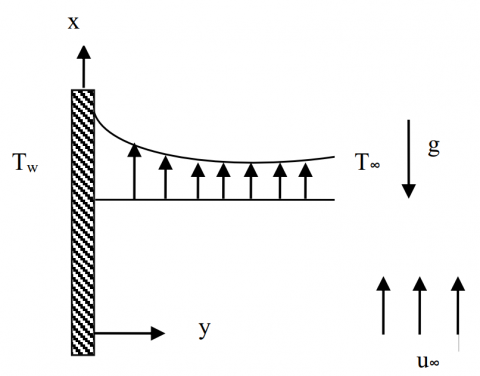Figure 1. Convection heat transfer over vertical flat plate embedded in porous medial.

The Boundary condition for slip and temperature jump

$\begin{array}{lc}y=0 \Rightarrow v=0 & T=T_{w}+D \frac{\partial T}{\partial y} \\ y=\infty \Rightarrow u=u_{\infty} & T=T_{\infty}\end{array}$    (4)

The partial differential equations (1-4) are transformed into ordinary differential equations using the following dimensionless variables:

$\eta=\frac{y}{x} P e_{x}^{1 / 2} \zeta^{-1}, \quad \zeta=\frac{1}{1+\left(R a_{x} / P e_{x}\right)^{1 / 2}}$

$\psi=\alpha_{m} P e_{x}^{1 / 2} f(s, \zeta) \zeta^{-1}, \quad \theta(\zeta, \eta)=\frac{T-T_{\infty}}{T-T_{\infty}}$     (5)

In the equations above, the steam function $\psi$ satisfies the continuity equation (1) with $u=\partial \psi / \partial y$ and $v=-\partial \psi / \partial x$. Finally, we can obtain the following system of dimensionless equations:

$f^{\prime}=(1-\zeta)^{2} \theta^{\prime}+\zeta^{2}$    (6)

$\theta^{\prime \prime}+\frac{1}{2} f \theta^{\prime}=0$   (7)

with the corresponding boundary conditions:

$\eta=0 \Rightarrow f(\zeta, 0)=0, \quad \theta=D^{*} \theta^{\prime}+1$

$\eta=\infty \Rightarrow f^{\prime}(\zeta, \infty)=\zeta^{2}, \quad \theta(\zeta, \infty)=0$     (8)

Where, $D^{*}=\frac{D P e_{x}^{1 / 2} \zeta^{-1}}{x\left(T_{w}-T_{\infty}\right)}$ is temperature jump parameter.

Some of the physical quantities of practical interest include the velocity components $u$ and $\mathcal{V}$ in the $x$ and $y$ directions, respectively. The local Nusselt number $N u_{x}=h x / k$ and the wall shear stress $\tau_{w}=\mu(\partial u / \partial y)$ . They are given by:

$u=u_{\infty} \zeta^{-2} f^{\prime}$   (9)

$v=-\left[\frac{1}{2 x} \alpha_{m} P e_{x}^{1 / 2}\left[f-\eta f^{\prime}\right]\right]$    (10)

$\left[P e_{x}^{1 / 2}+R a_{x}^{1 / 2}\right]^{-1} N u=-\theta^{\prime}(\zeta, 0)$      (11)

$\tau_{w}\left(x^{2} / \mu \alpha_{m}\right)\left(P e^{1 / 2}+R a^{1 / 2}\right)=f^{\prime \prime}(\zeta, 0)$   (12)

3. Numerical Solution

The ordinary differential equations (6) and (7) with boundary condition (8) are nonlinear coupled ordinary differential equations which have no closed form solution. Therefore, they must be solved numerically. First the second order ordinary differential equation is converted into a first order by substitutions $\theta^{\prime}=v$ , then by using by forward finite difference method

$\frac{\partial h}{\partial \eta}=\frac{h_{i+1}-h_{i}}{2 \Delta \eta}$    (13)

where $h$ is any dependent variable and $i$ is the node locations along the $\eta$ directions. A convergence criterion based on the relative difference between the current and previous iterations is employed. When this difference reaches 10-5, the solution is assumed to have converged and the iterative process is terminated.

The accuracy of the selected method was tested by comparing the results with those of Duwairi and Damseh . Table 1 shows a comparison between the Nusselt numbers at different convection parameter $\zeta$.

Table 1. Values of $\theta^{\prime}(\zeta, 0)$ compared to those obtained by Duwairi and Damseh 

 $\zeta$ Present study Duwairi and Damseh  0 -0.44353 -0.4429 0.1 -0.40341 -0.4062 0.5 -0.36155 -0.3611 0.7 -0.41992 -0.4170 0.9 -0.51408 -0.5089 1 -0.56952 -0.5439
4. Results and Discussion

The effects of temperature jump parameter on the natural, mixed and forced convection heat transfer rates are discussed below:

4.1 Natural Convection

Figures 2-4, show velocity, temperature, and shear stress profiles for natural convection $\zeta=0$ with different values of temperature jump parameter D*=0, 0.6, 1.5, zero value of D* means no temperature jump. It is noticed that the increasing of D* causes decrease in velocity and temperature at the surface and inside boundary layer, the temperature jump effect vanishes gradually far away from the surface, so the natural convection heat transfer rates are decreased as temperature jump increased parameter is increased, because of low momentum transfer rates inside the boundary layer. The  increasing of D* implies decreasing in shear stress with less effects at distance far from the surface with the boundary layer.

Physically, as the temperature jump parameter D* increases, the fluid near the surface of the plate and in the boundary layer will not sense the heating effects of the plate and less amount of heat will be transferred from the hot plate to the fluid and hence the velocity, wall shear stress, the temperature and the rates of heat transfer are decreased.

## 2.png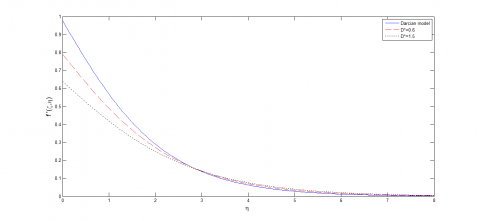Figure 2. Dimensionless velocity profiles for selected values of D*

## 3.png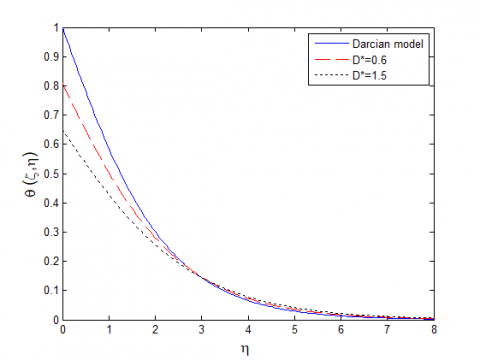Figure 3. Dimensionless temperature profiles for selected values of D*

## 4.pngFigure 4. Dimensionless skin friction profiles for selected values of D*

4.2 Mixed Convection

Figures (5-7) show velocity, temperature, and shear stress profiles for mixed convection heat transfer rates at selected value of $\zeta=0.5$ for different temperature jump parameter D*, zero value of D* means no temperature jump. It is noticed that the increasing of D* is accompanied with decrease in velocity and temperature at the surface of plate, going far from the surface, temperature jump effect vanishes gradually. The mixed heat transfer rates are consequently decreased due to insufficient momentum transfer between fluid layers. The shear stress is also decreased with increasing of D* values.

Again as the temperature jump parameter D* increases, the fluid near the surface of the plate and in the boundary layer will not sense the heating effects of the plate and less amount of heat will be transferred from the hot plate to the fluid and hence the velocity, wall shear stress, the temperature and the rates of heat transfer are decreased.

## 5.pngFigure 5. Dimensionless velocity profiles for selected values of D*

## 6.pngFigure 6. Dimensionless temperature profiles for selected values of D*

## 7.png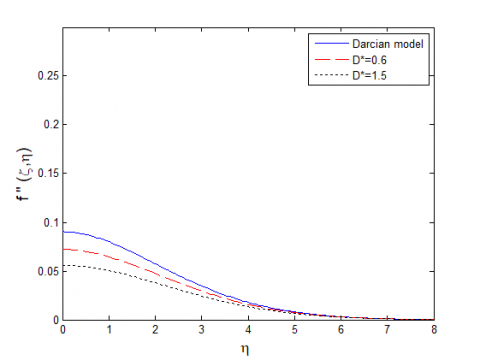Figure 7. Dimensionless skin friction profiles for selected values of D*

4.3 Forced Convection

Figures (8-10) show, velocity, temperature, and shear stress profile, for forced convection heat transfer $\zeta=1$, note that D* is zero means no temperature jump, it is observed that the velocity is constant for all D* value, thus, D* is not significant because of separating momentum form energy transfer inside boundary layer, while it has its effect on temperature at the surface and within boundary layer, where at lower temperature jump parameter D*, more heat transfer from surface to the fluid is noted, hence the temperature and the heat transfer rates are decreased in forced convection also.

## 8.pngFigure 8. Dimensionless velocity profiles for selected values of D*

## 9.png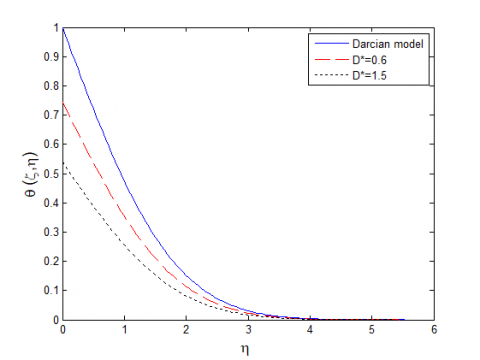Figure 9. Dimensionless temperature profiles for selected values of D*

## 10.png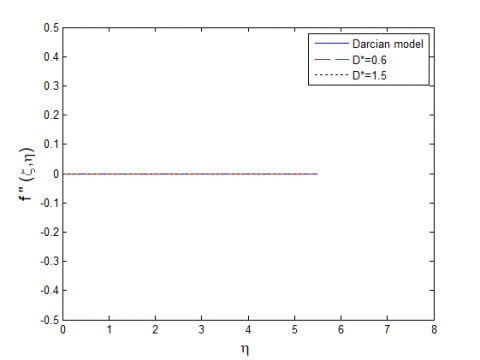Figure 10. Dimensionless skin friction profiles for selected values of D*

4.4 Nusselt Numbers and Shear Stresses

In figures (11-12), the increasing of  D* decreased values of Nusselt numbers and reduced heat transfer rates. Figure (13), shows the shear stress verses D* for different types of convection heat transfer, it can be noticed that the values of skin friction coefficient are decreased, this is due to small velocities inside boundary layer.

## 11.pngFigure 11. Local Nusselt number values for selected values of D*

## 12.pngFigure 12. Local Nusselt number values against D*

## 13.pngFigure 13. Dimensionless skin friction against D*

5. Conclusion

The effects of temperature jump on convection from vertical plate embedded in porous medium were studied numerically. The following results have been found:

(1) The increasing of temperature jump parameter decreased the velocities and temperatures near wall and inside boundary layers for natural and mixed convection heat transfer problems; this is due to insufficient momentum and energy transfer between the surface and the fluid. The temperature jump does not affect the velocity profiles for forced convection heat transfer problems.

(2) The heat transfer rates are decreased with increasing temperature jump parameter for natural, mixed and forced convection heat transfer problems; this is due to the slip effect of temperature near the wall.

(3) The shear stresses are decreases with increasing temperature jump parameter; this is due to small velocities inside boundary layers.

Nomenclature
 D Temperature jump factor D* Temperature jump parameter $f$ Dimensionless steam function g Gravitational acceleration, m/s2 K Permeability, m2 Nu Local Nusselt number, hx/k P Pressure, Pa Pe Local Peclet number, $u_{\infty} x / \alpha$ Ra Local Raylrigh number, $K g \beta\left(T_{w}-T_{\infty}\right) x / v \alpha$ $T_{\infty}$ Ambient temperature, K $T_{w}$ Wall temperature, K u,v Darcian velocity components in x and y directions. x Coordinate along the plate y Coordinate normal to the plate Greek Letters $\alpha_{m}$ Effective thermal diffusivity of the porous media $\beta_{T}$ Coefficient of thermal expansion, $\zeta$ Non-similarity parameter, $1 /\left[1+(R a / P e)^{1 / 2}\right]$ $\eta$ Pseudo-similarity variable $\theta$ Dimensionless temperature $\mu$ Dynamic viscosity $v$ Kinematic viscosity $\rho$ Fluid density $\tau_{w}$ Local wall shear stress $\psi$ Dimensional stream function Subscripts w Surface conditions $\infty$ Free stream condition
References

1. Bejan, A. and Poulikakos, D., The non-Darcy regime for vertical boundary layer natural convection in a porous medium. International Journal of Heat and Mass Transfer, 27, 717–722, 1984.

2. Chien, C.H. Chien, T.S. and Chen, C.K., Non-Darcy mixed convection along a nonisothermal vertical surface in porous media. Heat Transfer, 39, 1156-1164, 1996.

3. Y. Al-Badawi and H. M. Duwairi, MHD Natural convection in Iso-flux enclosures Filled with Porous Medium, Int. J. Heat & Technology, vol. 28, no. 2, pp. 89-93, 2010.

4. F. G. Shehadeh and H. M. Duwairi, MHD natural convection with Joule and viscous heating effect in Porous media-Filled enclosure, Int. J. Heat & Technology, vol. 28, no. 1, pp. 31-36, 2010.

5. James, D.F. and Davi, A.M., Flow at the interface of a model fibrous porous medium. Journal of Fluid Mechanics, 426, 47-72, 2001.

6. Cao, K. and Baker, J., Slip effects on mixed convective flow and heat transfer from a vertical plate. International Journal of Heat and Mass Transfer, 52, 3829–3841, 2009.

7. Yazdi, M.H. Abdullah, S. Hashim, I. and Nopiah, Z.M., Convection heat transfer of slip liquid flow past horizontal surface within the porous media at constant heat flux boundary conditions, Proceedings of the American Conference of Applied Mathematics, American-Math, 10, 27-29, 2010.

8. Bhattacharyya, B. Mukhopadhyay,S. and Layek, G., Steady boundary layer slip flow and heat transfer over a flat porous plate embedded in a porous media. Petroleum Science and Engineering, 78, 304–309, 2011.

9. Bhattacharyya, B., Slip effects on boundary layer flow and mass transfer with chemical reaction over a permeable flat porous plate in a porous media. Frontiers in Heat and Mass Transfer, 3, 2151-8629, 2012.

10. Miguel, A.F., Non-Darcy porous media flow in no-slip and slip regimes. Thermal science, 16(1), 167-176, 2012.

11. Jashim Uddin, Pop. I, and M.A.A.Ismail, A.I.MD. Free Convection Boundary Layer Flow of a Nanofluid from a Convectively Heated Vertical Plate with Linear Momentum Slip Boundary Condition. Sains Malaysiana, 41(11), 1475–1482, 2012.

12. Akhilesh, K. and Mishra. Mixed convection flow through a porous medium bounded by two vertical walls with slip boundary conditions. International Journal of Engineering Research & Technology, 2(3), 2013.

13. Njael, W.N.M. and Makinde, O.D., Combined effect of buoyancy force and Navier slip on MHD Flow of a Nano-fluid over a convectively heated vertical porous plate. The Scientific World Journal, 725643, 8, 2013.

14. Dwuairi, H.M. and Damseh, R.A., Effect of thermophoresis particle deposition on mixed convection  from vertical surfaces embedded in saturated porous medium. International journal of numerical methods for heat and fluid flow, 18 (2), 202-216, 2008.